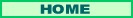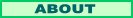µ Best Introduction to Tensors

Search

More Tech Stuff:

Indexing Books: Lessons in Language Computations

Client-Side Frame Manipulation Inside the Microsoft Internet Explorer Object Model with Visual Basic .NET

Replacing a PC power supply

Constructing a Mandelbrot Set Based Logo with Visual Basic.NET and Fireworks

Tensors are mathematical objects that can be used to represent real-world systems. Properly introduced, their basic nature is not hard to understand. Tensors have proven to be useful in many engineering contexts, in fluid dynamics, and for the General Theory of Relativity. Knowledge of tensor math (called tensor calculus or the absolute differential calculus) also is useful in the fields of financial analysis, machine understanding (artificial intelligence), and in the analysis of other complex systems.

Tensors are often introduced as being vectors on steroids. That is not an unfair generalization, but the way vectors are taught in high school often leads to misunderstandings when steroids are added.

Starting with the simplest case, we have scalars. These are single numbers that might represent readings from a scale. They are the numbers of ordinary arithmetic (they could also be complex numbers, or other math objects, but in this essay we'll stick to real numbers). Following high school algebra, we'll let x represent a number, but we will also add subscripts so that we can have lots of variables, rather than naming them a, b, x, y, z, etc. Variables are just variables, whatever symbol you use to represent them. A number of schemes are used for tensor variables, often dependent on the branch of engineering or science they are used for. Here I'll use a's with subscripts to keep the discussion as general as possible. For coordinate axes I'll use x's, with subscripts if necessary [the x,y,z axes of high school become the x1, x2, and x3 coordinate axes].

To show that we are working with a scalar, not just a number, we put the scalar in brackets, hence: [a].

We say scalars are one-dimensional. We also say scalars can be tensors of rank zero. Rank will be explained soon. Scalars are like individual lego pieces, or atoms: although simple, they are the basis of far more complex, far more interesting systems.

Since scalars are so simple, we can use them to introduce other topics important to tensor analysis: fields and coordinate systems. When we say a field we mean a space with objects at every point. If the objects are scalars, we have a scalar field. For instance, in two dimensions, for a plane, if there is a scalar value at each point, we have a two-dimensional scalar field. A physical example might be a surface, with the scalar values corresponding to temperatures at points on the surface.

Coordinate systems assign reference numbers to points in spaces. Most commonly, both for high-school math and for everyday use, we use the cartesian coordinate system of dimensions set at right angles to each other. But other coordinate systems simplify the math in certain engineering and science problems. The two-dimensional polar coordinate system is an example, as are cylindrical and spherical coordinates. Also we may find it convenient to use two or more different cartesian systems to solve a problem. In simple problems about motion for example, we might have a coordinate system we designate as stationary and a second coordinate system that moves with a set velocity along one of the axes of the first coordinate system (its origin moves).

Now, back to vectors. In high school these are presented as arrows, used in introductory physics to represent forces or velocities. Because they can represent a lot of varied things, for tensors it is best to think of vectors as ordered sets of numbers. For each dimension in the space under consideration vectors use one real number. We put the numbers in brackets, corresponding to the number of dimensions in the space. For a two dimensional plane the general vector can be represented as [a1 a2] and a specific vector example could be [1.2 3.7]. In a five dimensional space the vectors can be represented as [a1 a2 a3 a4 a5]. Vectors can also be represented as columns of numbers, which is conventional for some applications.

In a vector field each point in the space has a vector associated with it. In an experiment the vectors might be found by data readings. In math problems they are usually designated by equations that take coordinates into account. Note that the numbers [a1 a2] for a vector in a field do not represent the numbers of the coordinates you would get if you used the high-school arrow type vector starting from the base point. The numbers equate to the endpoint coordinates the vector would reach if it were at the origin. This makes sense if you think of the many objects a vector might represent, like forces. They don't really represent movement within the coordinate system, but do correspond to a direction in the coordinate system. They are non-spatial variables or specific data. Just like our scalar temperature readings are non-spatial.

What happens to vectors when we change coordinate systems? Usually the specific numbers representing the vector change. In a very simple example consider two separate cartesian coordinate systems, which have axes aligned, but in which the second system X has its origin at the point (5,0,0) of the first system, X. [It is more common to distinguish coordinate systems by having the second have a bar over its name, but underlining does just as well and is easier in HTML. It is also common to distinguish systems with apostrophes, as in X and X']. Suppose the vector [1,1,0] is located at the point (0,0,0) of system X. Then it is located at (5,0,0) of system X. If we transform the coordinates from X to X, the vector itself does not change, it remains [1,1,0]; just the coordinates of its position change.

With more complex changes in coordinate systems, both the numbers representing the vector might change as well as the coordinates of its position. Without worrying about the actual transformation formulas, lets call them T when going from X to X, and T when going from X to X. Similarly, in the X system we'll call our vector V, but the same vector (representing a real object, such as magnetic force) in the X system will be called V, because it probably has different actual numbers for [a1 a2]. Thus

V = T(V)

V = T(V)

Now, you have been dying to get to tensors. Surprise! You already have. It is said:

Vectors are tensors of rank 1.

But the beginning student is apt to misinterpret this statement, because it assumes you already know that tensors are not free standing objects. They always include transformation laws. So it should be stated:

Vectors with appropriate transformation laws for coordinate system changes are tensors of rank 1.

Before progressing, let's take a step back to scalars.

Scalars, with appropriate transformation laws for coordinate system changes, are tensors of rank 0.

Now, about rank, also called the order by some authors. The rank refers to how many dimensions an array has to have to represent the tensor. [a] is like a point, so scalars require 0 dimensions and have rank 0. [a1 a2 a3 a4 a5] is like a line, so it has 1 dimension, and vectors have rank 1.

The fun really begins when we start using tensors of rank 2, and so it is easy to forget that scalars and vectors can be tensors. Often, the word tensor is used only for tensors of rank 2 or greater.

Next: Tensors of Rank 2 (This project has been suspended for now)